• MATLAB带阻滤波器设计## ** %程序设计 %任务书中给出的要求为中心频率200Hz，带宽150Hz。 %故设上通带截止频率为110Hz，下通带截止频率290Hz，阻带上限频率140Hz，阻带下限频率260Hz。 %此处仅以Boxcar窗为示例，...
**
MATLAB带阻滤波器设计##
**
%程序设计
%任务书中给出的要求为中心频率200Hz，带宽150Hz。
%故设上通带截止频率为110Hz，下通带截止频率290Hz，阻带上限频率140Hz，阻带下限频率260Hz。
%此处仅以Boxcar窗为示例，其他窗函数的程序代码基本相同，
%只是在window=Boxcar(N)、N=ceil(1.8*pi/delta_w)两处作出各个窗函数相应的修改即可。
flp=490;
fhp=510;
fls=499;
fhs=501;
fs=2048;
wlp=2*pi*flp/fs;
whp=2*pi*fhp/fs;
wls=2*pi*fls/fs;
whs=2*pi*fhs/fs;
wc=[(wlp+wls)/(2*pi),(whp+whs)/(2*pi)];
delta1=wls-wlp;
delta2=whp-whs;
delta_w=min(delta1,delta2);
N=ceil(1.8*pi/delta_w);  %//不同的窗要选择系数不同//
N=N+rem(N,2);
n=0:N-1;
window=boxcar(N+1);  %//选择窗函数//
[h1,w]=freqz(window,1);
subplot(2,2,1)
stem(window,'.');
xlabel('n');
title('Boxcar窗函数');
subplot(2,2,2)
plot(w*fs/(2*pi),20*log(abs(h1)/abs(h1(1))));
grid;
xlabel('f/Hz');
ylabel('幅度(dB)');
title('Boxcar窗函数的频谱');
hn=fir1(N,wc,'stop',window);
[h2,w]=freqz(hn,1,512);

subplot(2,2,3)
stem(hn,'.');
xlabel('n');
ylabel('h(n)');
title('Boxcar窗函数的单位脉冲响应');
subplot(2,2,4)
plot(w*fs/(2*pi),20*log(abs(h2)/abs(h2(1))));
grid;
xlabel('f/Hz');
ylabel('幅度(dB)');
title('Boxcar带阻滤波器的幅频特性');



展开全文信号处理
• PAGE PAGE # / 7 基于的带通带阻滤波器设计实例 以下两个滤波器都是切比雪夫 I型数字滤波器不是巴特沃尔滤波器请使用者注意! 1.带通滤波器 (13) 滞通滤波 %吏用注意事项通带或阻带的截止频率与采样率的选取范围是不...
• ## matlab设计模拟带阻滤波器

万次阅读 热门讨论 2018-10-24 22:13:11
简单记录下在matlab上如何设计出模拟的带阻滤波器，包括：巴特沃斯滤波器、切比雪夫I型滤波器、切比雪夫II型滤波器、椭圆型滤波器。 %设计带阻滤波器 %巴特沃斯、切比雪夫I型、切比雪夫II型、椭圆型滤波器 clear ...
简单记录下在matlab上如何设计出模拟的带阻滤波器，包括：巴特沃斯滤波器、切比雪夫I型滤波器、切比雪夫II型滤波器、椭圆型滤波器。
%设计带阻滤波器
%巴特沃斯、切比雪夫I型、切比雪夫II型、椭圆型滤波器

clear all;

%wp和ws分别是通带和阻带的频率(截止频率)。当wp>ws时，为高通滤波器；当wp和ws为二元矢量时，为带通或带阻滤波器，这是求出的Wn也是二元矢量

%wp和ws为二元矢量
wp=[0.075*2*pi 0.2*2*pi];               %设置通带频率
ws=[0.1*2*pi 0.15*2*pi];                %设置阻带频率

Rp=1;                                   %设置通带波纹系数
Rs=20;                                  %设置阻带波纹系数

%巴特沃斯滤波器设计
[N,Wn]=buttord(wp,ws,Rp,Rs,'s');        %求巴特沃斯滤波器阶数，输出参数N代表满足设计要求的滤波器的最小阶数，Wn是等效低通滤波器的截止频率
%无论是高通、带通和带阻滤波器，在设计中最终都等效于一个截止频率为Wn的低通滤波器(我现在也不是很理解为啥是这样，毕竟我也是刚接触滤波器)
fprintf('巴特沃斯滤波器 N= %4d\n',N);    %显示滤波器阶数
[bb,ab]=butter(N,Wn,'stop','s');        %求巴特沃斯滤波器系数，即求传输函数的分子和分母的系数向量
W=0:0.01:2;                             %设置模拟频率
[Hb,wb]=freqs(bb,ab,W);                 %求巴特沃斯滤波器频率响应
plot(wb/pi,20*log10(abs(Hb)),'b');      %作图
hold on

%切比雪夫I型滤波器设计
[N,Wn]=cheb1ord(wp,ws,Rp,Rs,'s');       %求切比雪夫I型滤波器阶数
fprintf('切比雪夫I型滤波器 N= %4d\n',N); %显示滤波器阶数
[bc1,ac1]=cheby1(N,Rp,Wn,'stop','s');   %求切比雪夫I型滤波器系数，即求传输函数的分子和分母的系数向量
[Hc1,wc1]=freqs(bc1,ac1,W);             %求切比雪夫I型滤波器频率响应
plot(wc1/pi,20*log10(abs(Hc1)),'k');    %作图

%切比雪夫II型滤波器设计
[N,Wn]=cheb2ord(wp,ws,Rp,Rs,'s');       %求切比雪夫II型滤波器阶数
fprintf('切比雪夫II型滤波器 N= %4d\n',N);%显示滤波器阶数
[bc2,ac2]=cheby2(N,Rs,Wn,'stop','s');   %求切比雪夫II型滤波器系数，即求传输函数的分子和分母的系数向量
[Hc2,wc2]=freqs(bc2,ac2,W);             %求切比雪夫II型滤波器频率响应
plot(wc2/pi,20*log10(abs(Hc2)),'r');    %作图

%椭圆型滤波器设计
[N,Wn]=ellipord(wp,ws,Rp,Rs,'s');       %求椭圆型滤波器阶数
fprintf('椭圆型滤波器 N= %4d\n',N);      %显示滤波器阶数
[be,ae]=ellip(N,Rp,Rs,Wn,'stop','s');   %求椭圆型滤波器系数，即求传输函数的分子和分母的系数向量
[He,we]=freqs(be,ae,W);                 %求椭圆型滤波器频率响应
%作图
plot(we/pi,20*log10(abs(He)),'g');
axis([0 max(we/pi) -30 2]);
legend('巴特沃斯滤波器','切比雪夫I型滤波器','切比雪夫II型滤波器','椭圆型滤波器');
xlabel('角频率{\omega}/{\pi}');
ylabel('幅值/dB');
line([0 max(we/pi)],[-20 -20],'color','k','linestyle','--');
line([0 max(we/pi)],[-1 -1],'color','k','linestyle','--');
line([0.15 0.15],[-30 2],'color','k','linestyle','--');
line([0.4 0.4],[-30 2],'color','k','linestyle','--');

运行结果如下：
巴特沃斯滤波器 N=    4
切比雪夫I型滤波器 N=    3
切比雪夫II型滤波器 N=    3
椭圆型滤波器 N=    2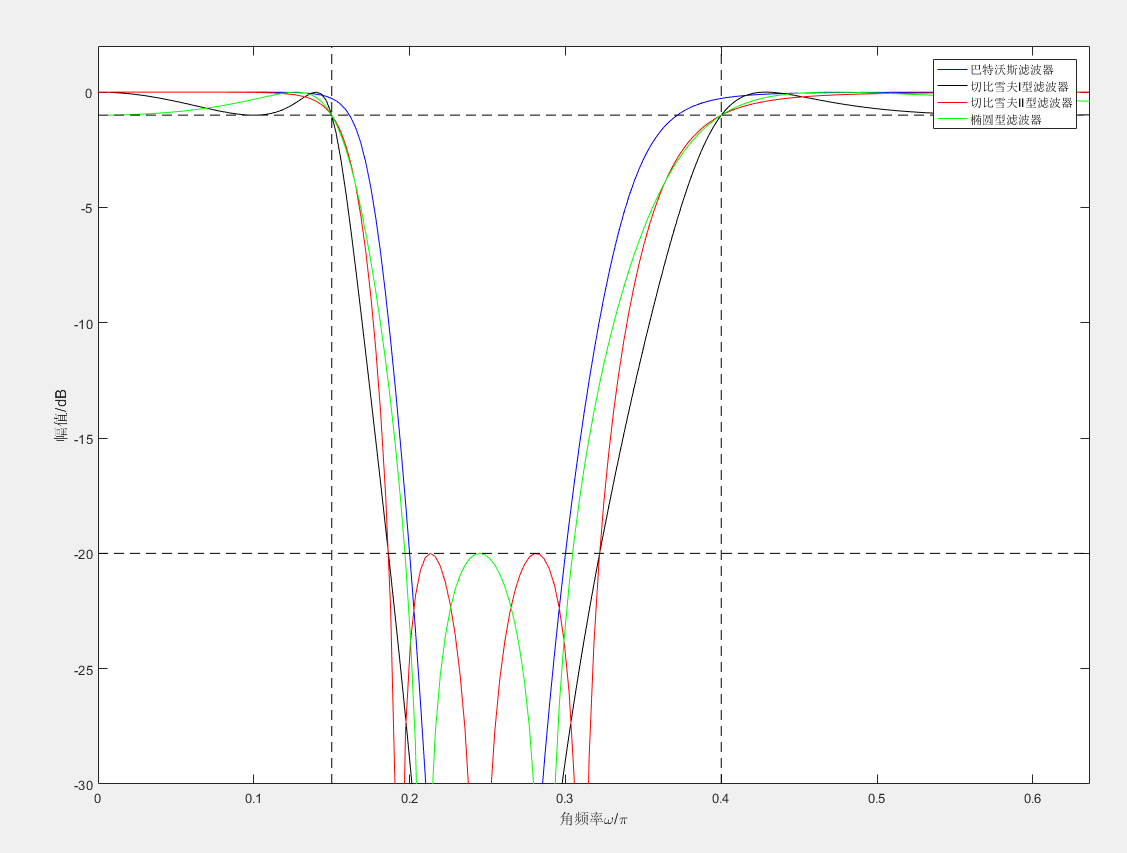展开全文• 1、设计中心频率为200Hz，带宽为150Hz的模拟带阻滤波器； 2、独立编写程序实现
• [例4-4] 试设计一个满足下列技术指标的BW型模拟带阻滤波器. wp1 = 6 rad/s, wp2 = 13 rad/s, ws1 = 9rad/s, ws2 = 11 rad/s, Ap <= 1dB, As >= 10dB. [问题分析] (1)根据带阻滤波器的阻带上下截频, 确定...
试设计一个满足下列技术指标的BW型模拟带阻滤波器.

wp1 = 6 rad/s, wp2 = 13 rad/s, ws1 = 9rad/s, ws2 = 11 rad/s, Ap <= 1dB, As >= 10dB.

[设计思路分析]

(1)根据带阻滤波器的阻带上下截频, 确定带阻滤波器的阻带宽度B和带阻滤波器的中心频率w0,

其中 B=ws2-ws1, wo^2=ws2·ws1;

(2)确定原型低通滤波器的通带截频wp'、阻带截频ws':

wp1' = (B·wp1) / (-wp1^2 + w0^2);

wp2' = (B·wp2) / (-wp2^2 + wo^2);

wp' = max{ |wp1'|, |wp2'| };

ws' = 1.

(3)设计技术指标为wp'、ws', Ap <= 1dB, As >= 10dB 的BW型模拟低通滤波器HL(s'):

由BW型模拟低通滤波器关于N的不等式可计算出N的值, 进而可计算出wc'的值.

这里经过较为复杂的计算, 可得出待设计模拟低通滤波器的系统函数HL(s').

(4)由复频率转换将原型低通滤波器HL(s')转换为带阻滤波器HBS(s).

[设计代码]

% 设计BW型模拟带阻滤波器
% 技术指标
wp1 = 6; wp2 = 13; ws1 = 9; ws2 = 11; Ap = 1; As = 10;
% (1)根据带阻滤波器的阻带上下截频, 确定带阻滤波器的阻带宽度B和带阻滤波器的中心频率w0
B = ws2 - ws1;
w0 = sqrt(ws2 * ws1);
% (2)确定原型低通滤波器的通带截频wp'、阻带截频ws'
wp1_ = (B * wp1) / (- wp1 * wp1 + w0 * w0);
wp2_ = (B * wp2) / (- wp2 * wp2 + w0 * w0);
if abs(wp1_) > abs(wp2_)
wp_ = abs(wp1_);
else
wp_ = abs(wp2_);
end
% 原型低通滤波器阻带截频取1
ws_ = 1;
% (3)设计技术指标为wp'、ws', Ap <= 1dB, As >= 10dB 的BW型模拟低通滤波器HL(s')
[N ,wc_] = buttord(wp_, ws_, Ap, As, 's');
[num, den] = butter(N, wc_, 's');
% (4)由复频率转换将原型低通滤波器HL(s')转换为带阻滤波器HBS(s)
[numt, dent] = lp2bs(num, den, w0, B);
% (5)画出带阻滤波器的增益响应
% 在[0, 20]区间上设置个3000个采样点
w = linspace(0, 20, 3000);
% freqs()返回一个模拟滤波器H(jw)的复频域响应(Laplace格式)
% freqs(num, den, w) -> 根据系数向量num, den计算并返回模拟滤波器的复频域响应H(s)
% 角频率w确定了输入的实向量, 因此必须包含至少一个频率点
h = freqs(numt, dent, w);
% 绘制所设计带阻滤波器的增益响应曲线
plot(w, 20 * log10(abs(h)));
% 输出所设计滤波器的重要参考指标
f = [wp1, ws1, ws2, wp2];
h = freqs(numt, dent, f);
fprintf('Ap1 = %.4f\n', -20 * log10(abs(h(1))));
fprintf('As1 = %.4f\n', -20 * log10(abs(h(2))));
fprintf('As2 = %.4f\n', -20 * log10(abs(h(3))));
fprintf('Ap2 = %.4f\n', -20 * log10(abs(h(4))));

[运行结果]

由实际仿真结果可知, Ap1 = 0.0511dB <= 1dB, As1 = 10dB, As2 = 10dB, Ap2 = 0.6867dB, 由此可见所设计模拟带阻滤波器满足技术指标.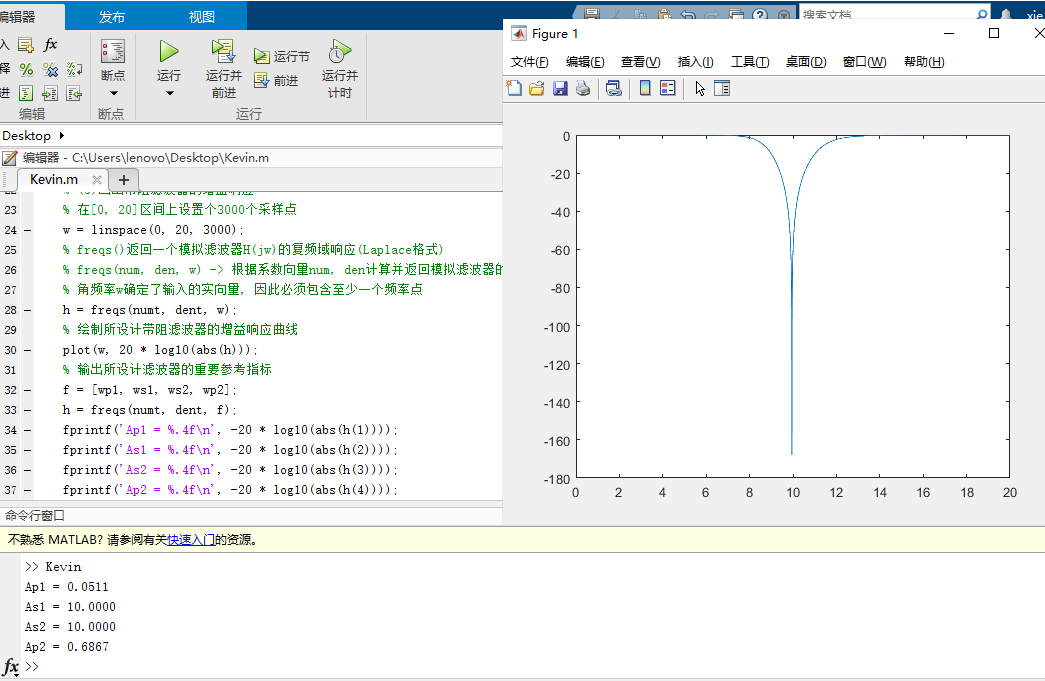展开全文信号分析与处理
• 基于matlab的FIR低通_高通_带通_带阻滤波器设计
• 北京师范大学 课程设计报告 课程名称 DSP 设计名称 FIR 低通高通带通和带阻数字滤波器设计 姓 名 学 号: 班 级 指导教师 起止日期 课程设计任务书 学生班级 设计名称 起止日期 学生姓名 学号 FIR 低通高通带通和...
• ; margin-right:0pt">求基于MATLAB的IIR数字带阻滤波器设计与实现的代码</p>
• 原始音频信号受到一正弦噪声（幅度0.1、频率1000Hz）的污染，请使用MATLAB设计一个带阻滤波器，滤除正弦噪声信号。 根据题目的要求，思路非常清晰，使用MATLAB完成设计任务的总体思路可按照以下四步进行: 1、...
• 北京师范大学 课 程 设 计 报 告 课程名称 DSP 设计名称 FIR 低通高通带通和带阻数字滤波器设计 姓 名 学 号: 班 级 指导教师 起止日期 课 程 设 计 任 务 书 精选文档 学生班级 学生姓名 学号 设计名称 FIR 低通...
• 有关DSP的带阻滤波器毕业设计，用了MATLAB设计其中的指标，并给出DSP的硬件电路设计和原理图
• 有频率为5Hz、15Hz、30Hz的叠加余弦信号，设计低通滤波器保留5Hz的频率分量，设计高通滤波器保留30Hz的频率分量，设计带通滤波器保留20Hz的频率分量，设计带阻滤波器滤除5Hz和30Hz的频率分量。 一、pandas是什么？ ...
文章目录问题提出一、低通滤波器1.保留5Hz2.运行结果二、高通滤波器1.保留30Hz2.运行结果三、带通滤波器1.保留20Hz2.运行结果四、带阻滤波器1.滤除5Hz和30Hz2.运行结果最后

问题提出
有频率为5Hz、15Hz、30Hz的叠加余弦信号，进行如下设计：
（1）设计低通滤波器保留5Hz的频率分量；
（2）设计高通滤波器保留30Hz的频率分量；
（3）设计带通滤波器保留20Hz的频率分量；
（4）设计带阻滤波器滤除5Hz和30Hz的频率分量。

一、低通滤波器
1.保留5Hz
clear
clc
f1=5;%第一个点频信号分量频率
f2=15;%第二个点频信号分量频率
f3=30;%第三个点频信号分量频率
fs=150;%采样率
T=2;%时宽
B=10;%FIR截止频率
n=round(T*fs);%采样点个数
t=linspace(0,T,n);
y=cos(2*pi*f1*t)+cos(2*pi*f2*t)+cos(2*pi*f3*t);%叠加信号
figure;
subplot(221)
plot(t,y);
title('原始信号时域');
xlabel('t/s');
ylabel('幅度');
fft_y=fftshift(fft(y));%将fft结果以fs/2为中心左右互换
f=linspace(-fs/2,fs/2,n);
subplot(222)
plot(f,abs(fft_y));
title('原始信号频谱');
xlabel('f/Hz');
ylabel('幅度');
axis([ 0 50 0 100]);
b=fir1(80, B/(fs/2),'low'); %低通
y_after_fir=filter(b,1,y);%
subplot(223)
plot(t,y_after_fir);
title('滤波后信号时域');
xlabel('t/s');
ylabel('幅度');
fft_y1=fftshift(fft(y_after_fir));%将fft结果以fs/2为中心左右互换
f=linspace(-fs/2,fs/2,n);
subplot(224)
plot(f,abs(fft_y1));
title('滤波后信号频谱');
xlabel('f/Hz');
ylabel('幅度');
axis([ 0 50 0 100]);
figure;
freqz(b);%数字滤波器频率响应

2.运行结果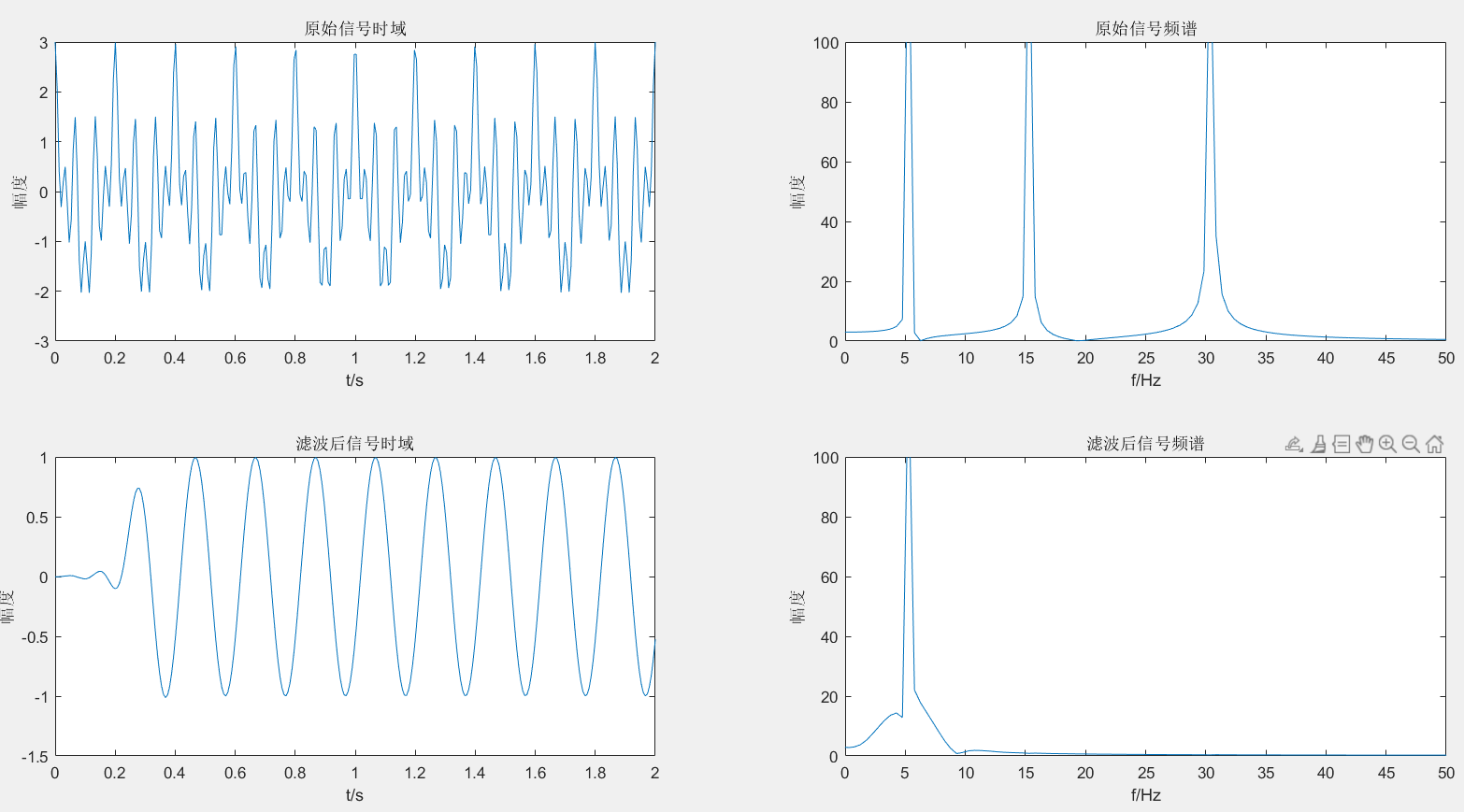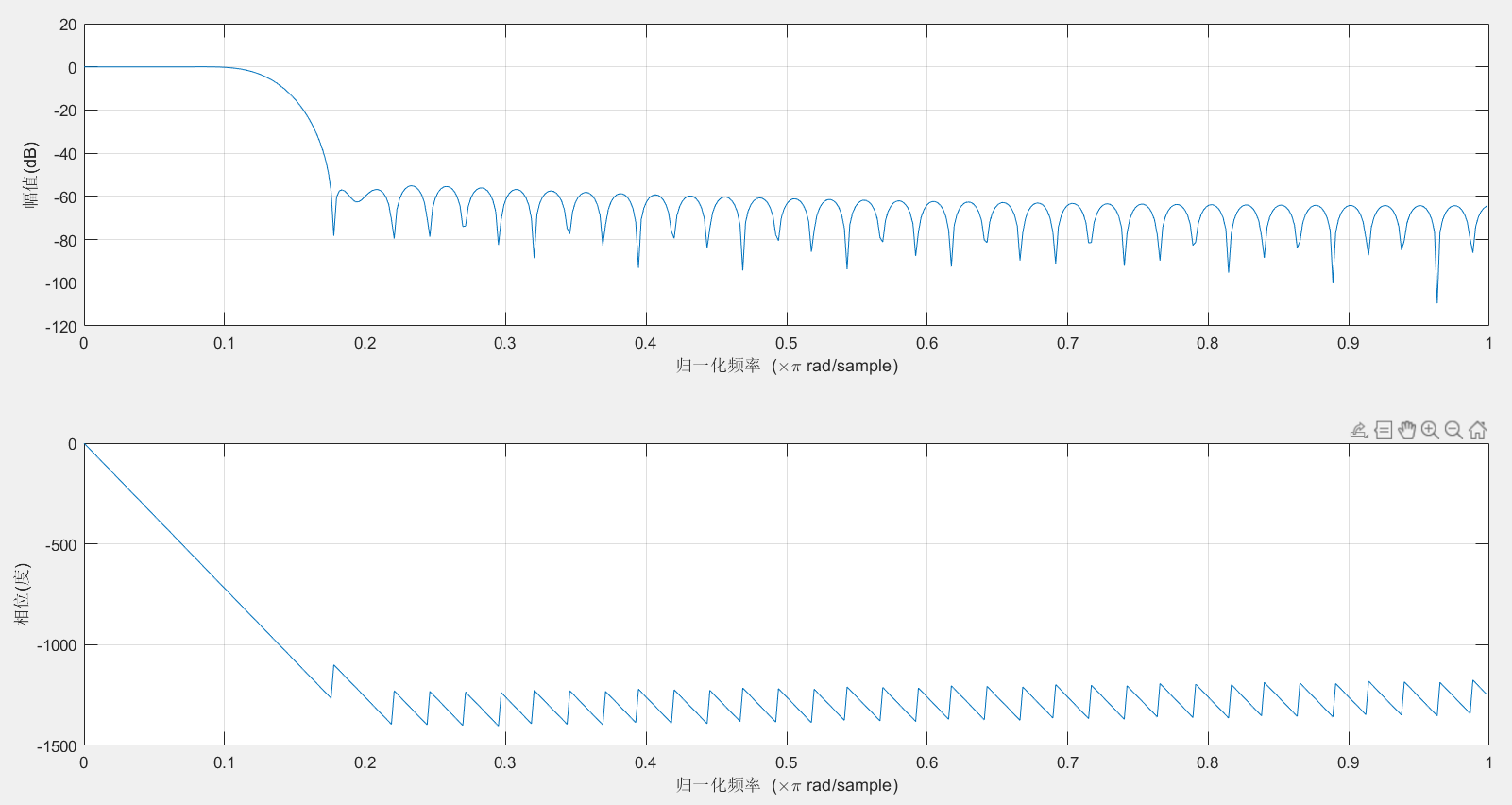二、高通滤波器
1.保留30Hz
clear
clc
f1=5;%第一个点频信号分量频率
f2=15;%第二个点频信号分量频率
f3=30;%第三个点频信号分量频率
fs=150;%采样率
T=2;%时宽
B=25;%FIR截止频率
n=round(T*fs);%采样点个数
t=linspace(0,T,n);
y=cos(2*pi*f1*t)+cos(2*pi*f2*t)+cos(2*pi*f3*t);
figure;
subplot(221)
plot(t,y);
title('原始信号时域');
xlabel('t/s');
ylabel('幅度');
fft_y=fftshift(fft(y));
f=linspace(-fs/2,fs/2,n);
subplot(222)
plot(f,abs(fft_y));
title('原始信号频谱');
xlabel('f/Hz');
ylabel('幅度');
axis([ 0 50 0 100]);
b=fir1(80, B/(fs/2),'high'); %高通
y_after_fir=filter(b,1,y);
subplot(223)
plot(t,y_after_fir);
title('滤波后信号时域');
xlabel('t/s');
ylabel('幅度');
fft_y1=fftshift(fft(y_after_fir));
f=linspace(-fs/2,fs/2,n);
subplot(224)
plot(f,abs(fft_y1));
title('滤波后信号频谱');
xlabel('f/Hz');
ylabel('幅度');
axis([ 0 50 0 100]);
figure;
freqz(b);%数字滤波器频率响应

2.运行结果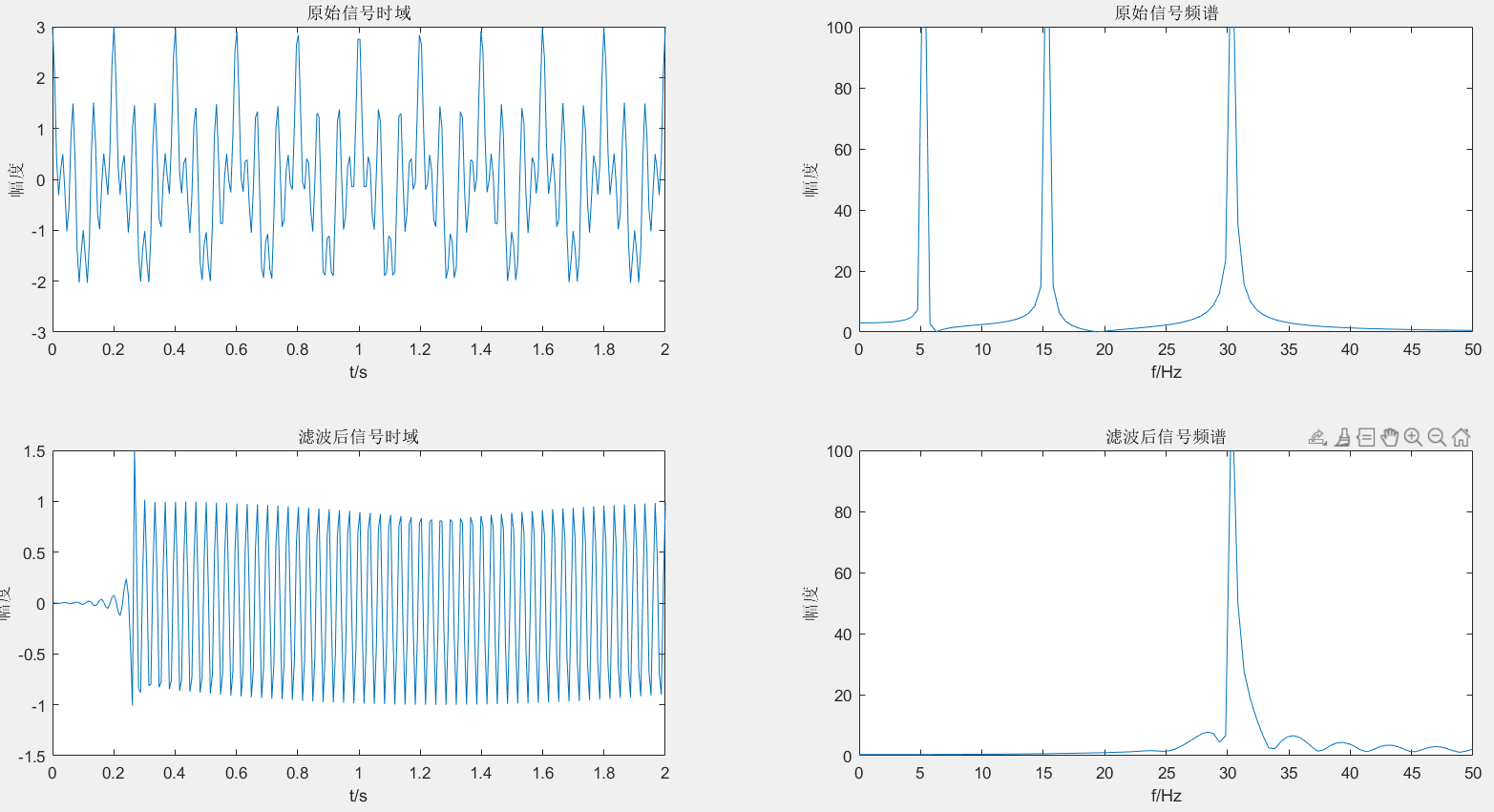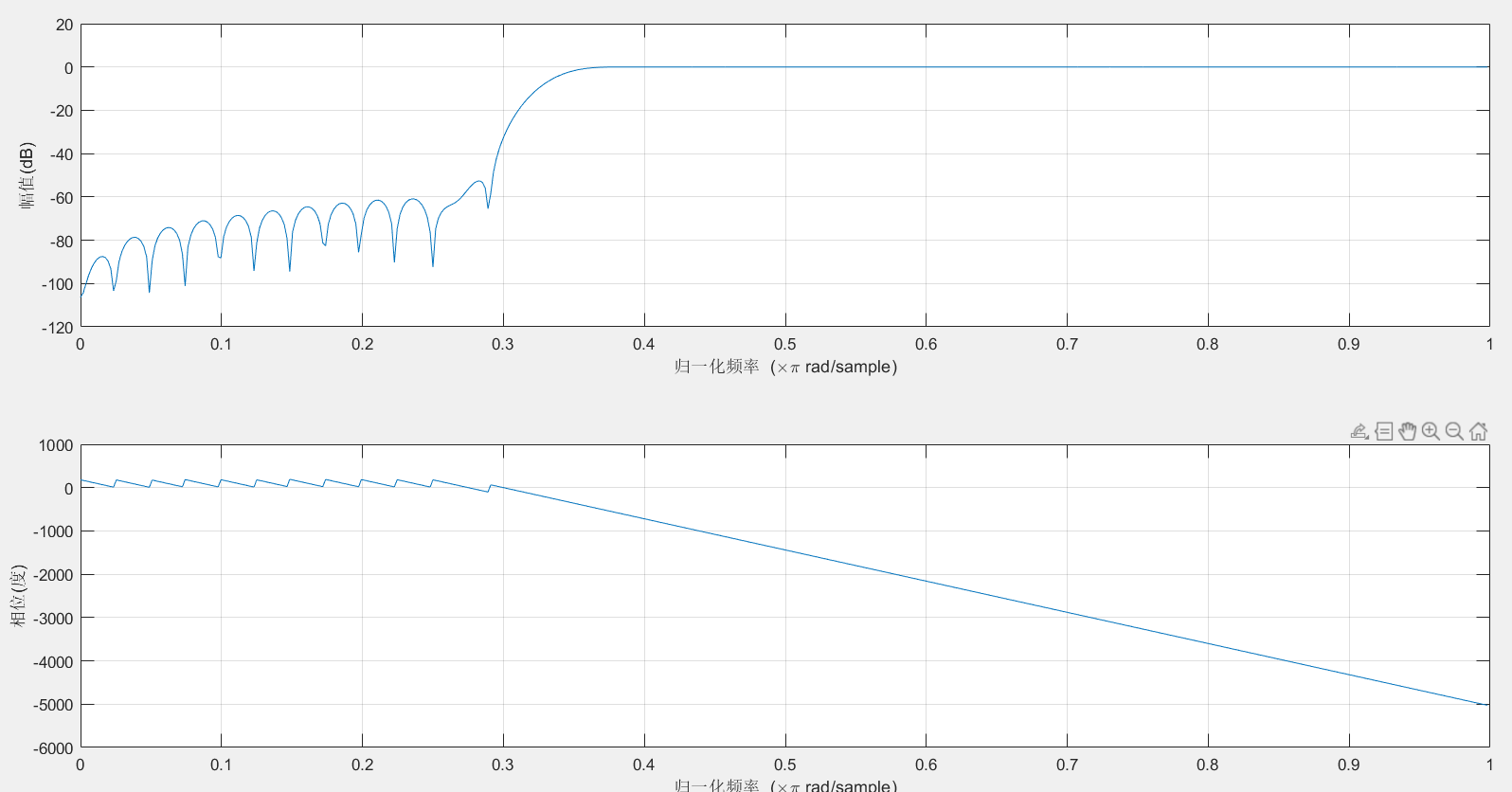三、带通滤波器
1.保留20Hz
clear
clc
f1=5;%第一个点频信号分量频率
f2=15;%第二个点频信号分量频率
f3=30;%第三个点频信号分量频率
fc1=10;
fc2=25;
fs=150;%采样率
[n,Wn,beta,ftype]=kaiserord([7 13 17 23] ,[0 1 0], [0.01 0.01 0.01] ,100) ;
w1=2*fc1/fs; w2=2*fc2/fs ;
window=kaiser(n+1 ,beta) ;%使用kaiser窗函数
b=fir1 (n, [w1 w2],window) ;%使用标准频率响应的加窗设计函数fir1
T=2;%时宽
n=round(T*fs);%采样点个数
t=linspace(0,T,n);
s = cos(2*pi*t*f1) +cos (2*pi*t*f2) +cos (2*pi*t*f3) ;
sf = filter (b,1,s) ;%对信号s进行滤波
f=linspace(-fs/2,fs/2,n);
S=fftshift(fft(s)) ;
SF=fftshift(fft(sf)) ;
figure
subplot(2,2,1) ;
plot(t,s)
%画出时域内的号
subplot(2,2,3) ;
plot(t,sf)
%画出时域内的信号
subplot(2,2,2) ;
f1=abs(S);
f2=abs(SF);
plot(f,f1) ; %画出频域内的信号
title('原始信号频谱');
axis([ 0 50 0 100]);
subplot(2,2,4) ;
plot(f,f2);%画出频域内的信号
title('滤波后信号频谱');
axis([ 0 50 0 100]);
figure;
freqz (b,1,512) ;%数字滤波器频率响应

2.运行结果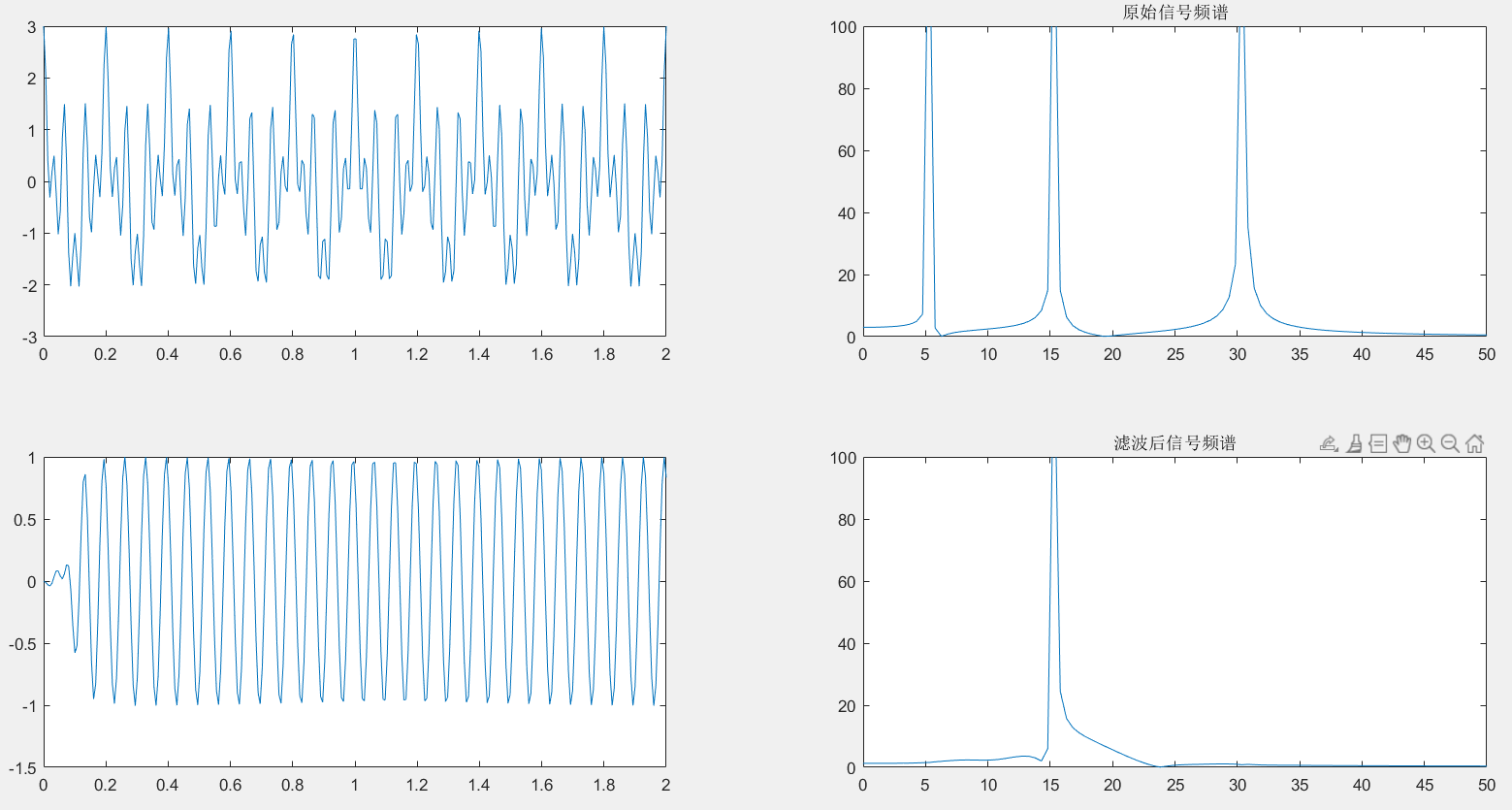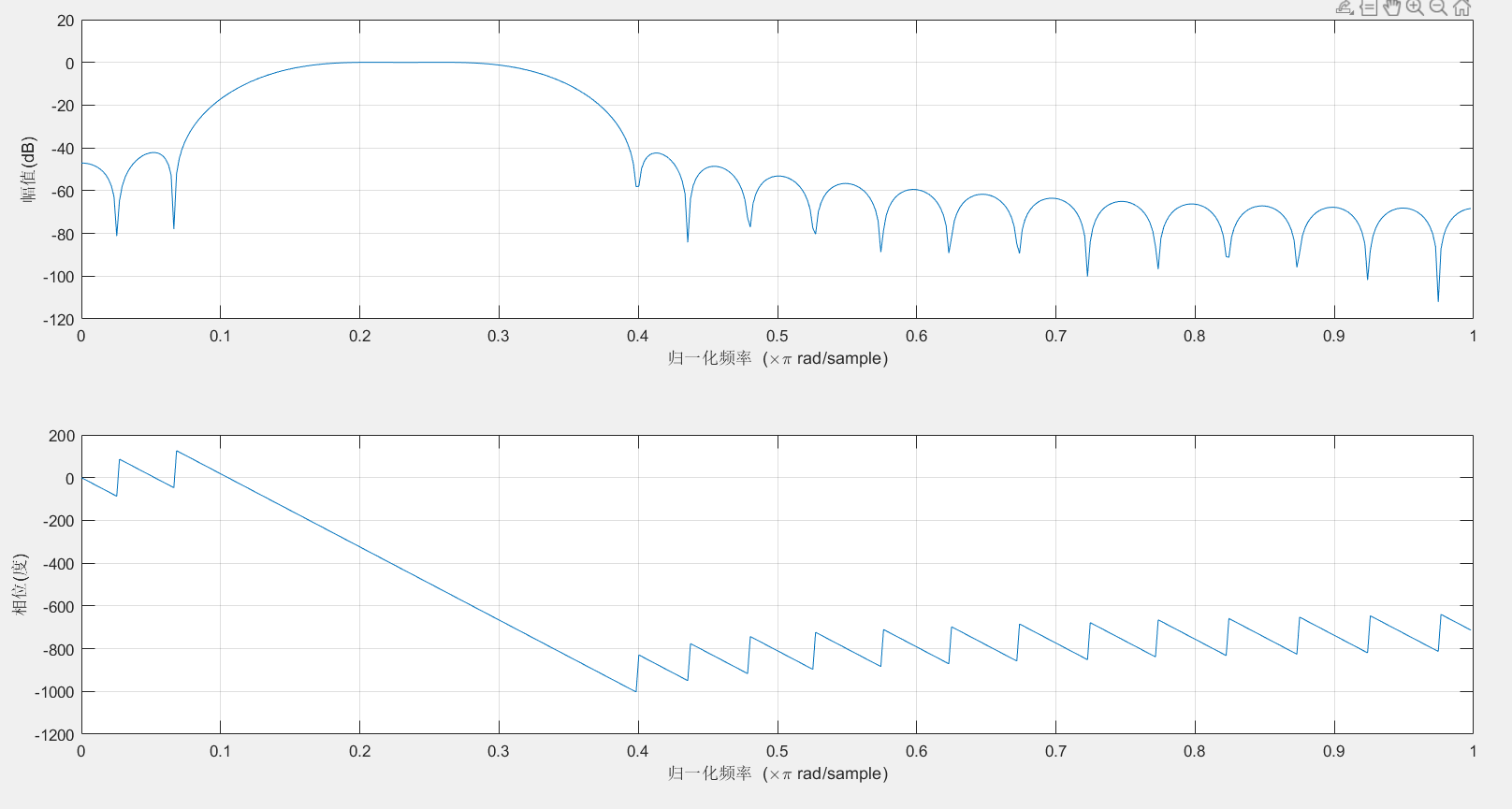四、带阻滤波器
1.滤除5Hz和30Hz
clear
clc
f1=5;%第一个点频信号分量频率
f2=15;%第二个点频信号分量频率
f3=30;%第三个点频信号分量频率
fs=150;
T=2;%时宽
n=round(T*fs);%采样点个数
t=linspace(0,T,n);
y=cos(2*pi*f1*t)+cos(2*pi*f2*t)+cos(2*pi*f3*t);
flp=0;
fhp=30;
fls=15;
fhs=25;
wlp=2*pi*flp/fs;
whp=2*pi* fhp/fs;
wls=2*pi*fls/fs;
whs=2*pi* fhs/fs;
wc=[(wlp+wls)/(2* pi),(whp+whs)/(2*pi)];
delta1=wls-wlp;
delta2=whp-whs;
delta_w=min(delta1 ,delta2);
N=ceil(1.8*pi/delta_w); %不同的窗要选择系数不同
N=N+rem(N,2);
window=boxcar(N+1); %选择窗函数
b=fir1 (28, wc,window) ;
ylb=filter(b,1,y);
f=linspace(-fs/2,fs/2,n);
y1=fftshift(fft(y));
ylb1=fftshift(fft(ylb)) ;
figure(1)
subplot(221)
plot(t,y)
subplot(222)
y1=abs(y1);
plot(f,y1)
title('原始信号频谱');
axis([ 0 50 0 100]);
subplot(223)
plot(t,ylb)
subplot(224)
ylb1=abs(ylb1);
plot(f,ylb1)
title('滤波后信号频谱');
axis([ 0 50 0 100]);
figure
freqz (b,1,512) ;%数字滤波器频率响应


2.运行结果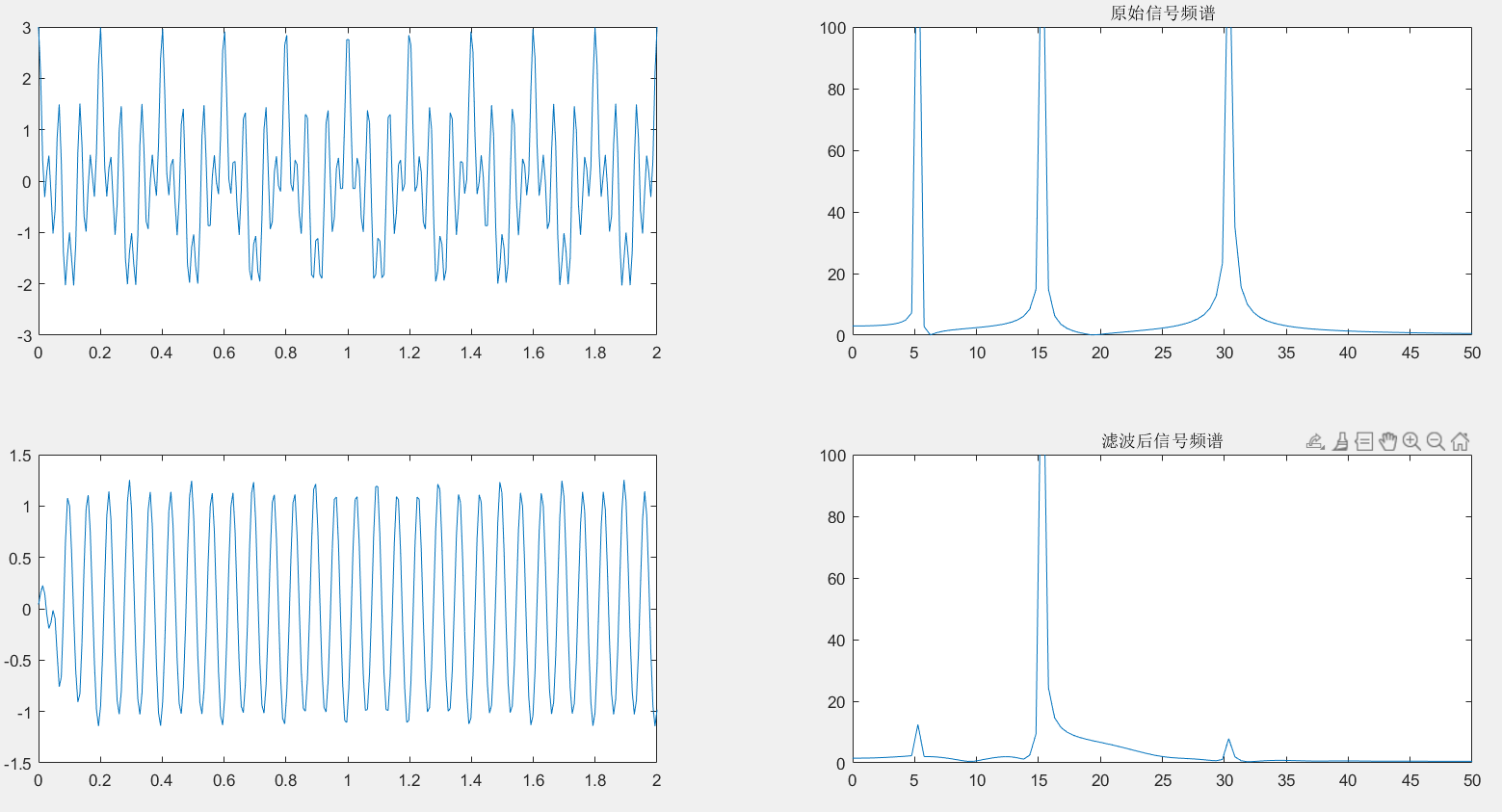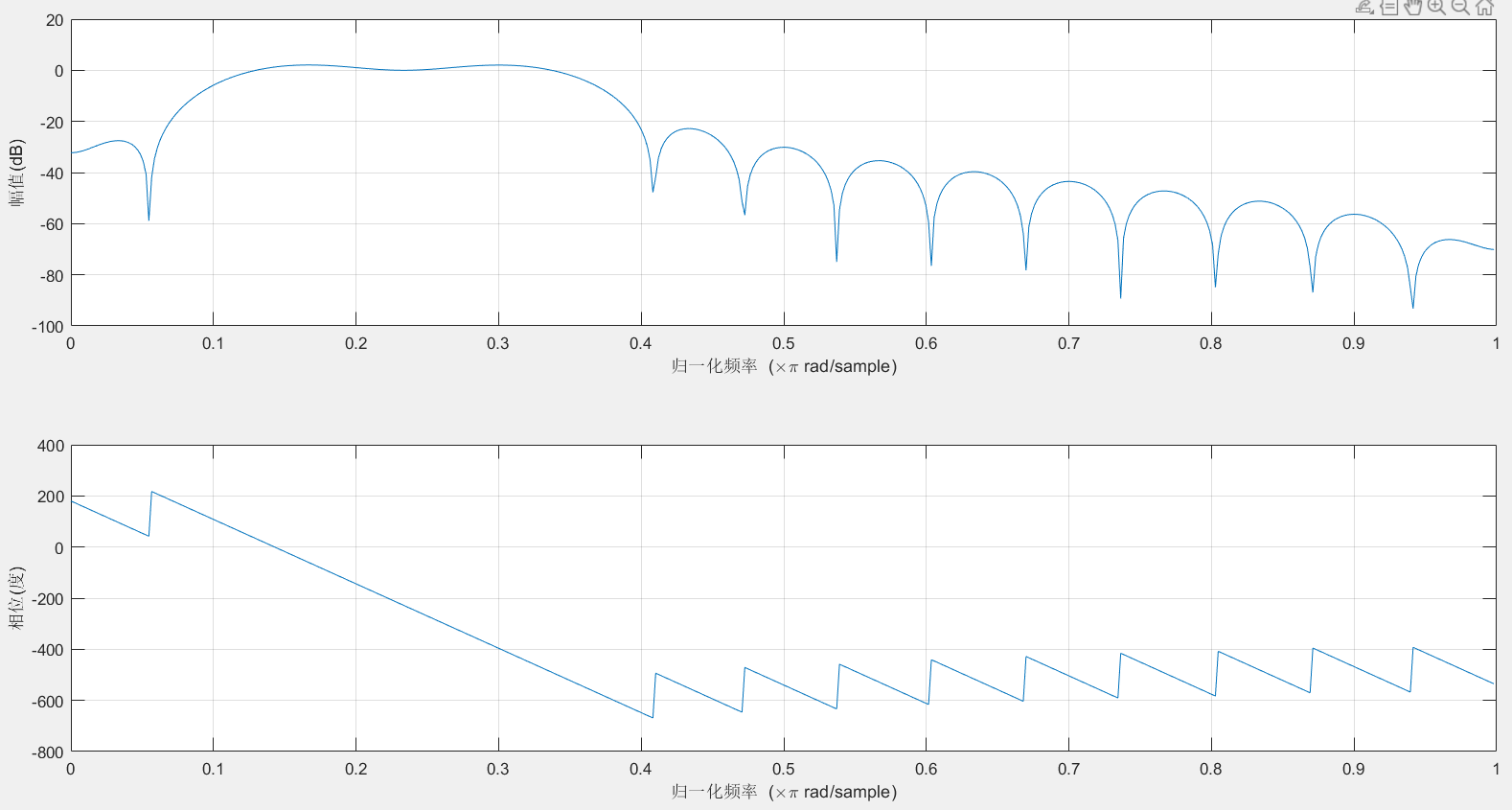最后
不定期发布一些matlab设计内容,敬请期待。包括但不限于如下内容:信号处理、通信仿真、gui设计、matlab appdesigner，simulink仿真。有任何有关MATLAB的问题可加QQ：2802009708进行咨询。或扫码进行添加。展开全文数字信号处理 filter
• 3）lp2hp，lp2bp，lp2bs可以完成低通滤波器到高通、带通、带阻滤波器的转换；4)使用bilinear可以对模拟滤波器进行双线性变换，求得数字滤波器的传输函数系数；5)利用impinvar可以完成脉冲响应不变法的模拟滤波器到...
• [Matlab]椭圆滤波器设计：低通、高通、带通和带阻-------（4） ​ 椭圆滤波器（Elliptic filter）又称考尔滤波器（Cauer filter），是在通带和阻带等波纹的一种滤波器。椭圆滤波器相比其他类型的滤波器，在阶数相同...数字信号处理
• 使用 MATLAB 设计 ISE中 FIR 滤波器系数的方法 1打开 MATLAB在命令行窗口输入 fdatool 打开Filter Designer & Analysis Tool 工具如下图所示 2由于 FPGA中滤波器的系数需要为整数 因此需要在此处将系数设置为 Fixed ...
• 首先来熟悉低通滤波的图，低的频率可以通过，高的频率不行。 空间域图像和频域图 分别为带通滤波器设计图，处理后的空间域图像，处理后的频域图 带通滤波器的设计
• 已经答辩通过的程序。保证可用。 分别是两个调用函数与一个主函数。 含验证程序。
• 通过MATALAB实现带阻滤波器设计设计中心频率为200Hz，带宽为150Hz的模拟带阻滤波器。通过利用MATLAB编程，并进行仿真，验证所编程序的正确性
• 陷波器(带阻滤波器)的实现困扰了我很久，这种滤波器在电力电子电源里面，算是比较高级的算法，能实现的人都不是菜鸟。单相逆变器中间母线电压存在二次波动，电压的二次波动导致电压外环输出也含有二次分量，如果不对...
• 椭圆滤波器（Elliptic filter）又称考尔滤波器（Cauer filter）： 这是在通带和阻带等波纹的一种滤波器。 椭圆滤波器相比其他类型的滤波器，在阶数相同的条件下有着最小的通带和阻带波动。 它在通带和阻带的...
• title(巴特沃斯低通滤波器"); xlabel(频率(HZ) );ylabel(耗损 (dB) );grid on; yd=filter(Bz,Az,y_ z); ydd=fft(yd,n); f=fs*(0:n/2-1)/n; figure(4); subplot(2,1,1 );plot(yd); title("滤波后信号时域波形); ......

# matlab带阻滤波器设计matlab 订阅